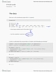# Textbook Guide Statistical Sciences: Frequency Distribution, Minitab, Probability Distribution

200 views8 pages
School
Course
Professorgoducksgo and 36820 others unlocked23
Verified Note
23 documents

## Document Summary

Normal distribution is the most commonly used distribution among all probability distribution. Normal probability distribution has many practical applications, for example, where the random variables are human characteristics such as weight, height, iq scores, length and speed. It must be remembered that normal does not mean the general type of distribution to be expected. Rather normal means the type that frequency distribution of certain variable tend towards normal. Bell-shaped normal curve has a single peak; thus, this is unimodal. The mean of the normal distribution lies at the centre of the normal curve, which is also the median and mode of the distribution. Normal probability distribution is symmetrical around a vertical line erected at the mean. Two tails of the normal curve (left-tail and right-tail) extend indefinitely but never touch the horizontal axis. Total area under the normal curve remains 1. Area under the normal curve specifies the probabilities for the normal random variable.

## Get access